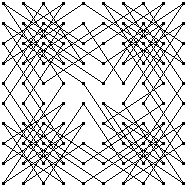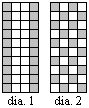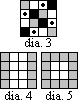This header plots the critical line of the Riemann Zeta Function.  A complete understanding wins a \$1,000,000 prize.
 . . .Main Links Orders Post Next Page Next + 10
LEAPERS (Chess Knights and the like)I intend to place a leaper-tour solving applet at this location.  For now, here is a small article I wrote.  I intended to expand this into my Master's Thesis, but Donald Knuth wrote an excellent article about leapers that made any expansion a moot point.

In February 1956, M Apsimon posed the question: how many cyclically symmetric knight tours on a board 10x10?  In the 1970's, W H Cozens reiterated the question in The Mathematical Gazette, and published the tours below.  More recently, Donald Knuth found that exactly 2,432,932 knight's tours are unchanged by 180-degree rotation of the chessboard.  It seems the problem from 1956 might now be answerable.1956 question - how many of these are there?
Patent 694038 (1902) by W E Stubbs patented a knights tour on a 6x6 board.  See GP Jelliss's page for the history of this problem. The Stubbs puzzle used a pegboard and pegs joined with cord.  To solve the puzzle, all the lengths of cord between the pegs had to be taut.  Suppose that you had a 5x5 grid of pegs.  Some holes are already filled, perhaps.  With 15-25 pegs, is there a series of lengths so that the pegs can only be put away tautly in a unique way?

In the March 1973 issue of Games and Puzzles, Robin Merson found a non-crossing knight's tour on an 11x11 board with a length of 74 moves.  He found a longer non-crossing tour on a board with a smaller area.  Can you find it?Roger Phillips has found a smaller board with a longer uncrossing tour.  A 10x12 board allows for a length 75 tour.  I've moved the material from a few weeks ago to the Leapers page.Juha Saukkola wondered what the longest possible Knightrider tour might be.  A knightrider moves like a knight, by may keep going in a straight line.  Here is his answer.  What is the fewest number of moves for a knightrider to tour the chessboard?Advanced Computing Technology has a program that solves large Leaper problems.

Keyed Tours.  Each path starts at the top left corner, and starts cycling through the KEY over and over again.  It will always take the direction suggested by the key IF a complete closed tour would still be possible.  There are three parts to the key.
1.  A direction key.  Below, a simple 1=N 2=E 3=S 4=W is used.  The eight paths of a knight could be labeled, or something more complicated.
2.  A grid.  Below, a 6x6 grid is used.  The grid could be multidimensional, or hexagonal, or even a grid of chaos tiles.
3.  A code.  Each code will produce a unique path.  On a 50x50 grid, what path would result from a 12345678 code from a knight?CONSTANT LENGTH TOURS  by Ed Pegg Jr.

Leonhard Euler was the first to find a closed tour of a chessboard by a knight.  Other pieces, more exotic, exist in the realm of Fairy Chess.  Until now, their tour potential hasnt been studied.  Ill start with definitions, most of them standard Graph Theory terms.

Point (vertex, node, junction, cell)
Line (edge, arc, branch)
Two points are adjacent (linked) if they are joined by a line.
A loop links a point to itself.  [None of the graphs in this paper contain loops.]
A line is incident to the points it joins.
The degree of a point is the number of lines incident to it.
Lattice - a graph where all points are midpoints of tiles in a regular tiling. [Ill deal with square tilings.]
A graph is connected if there exists a sequence of points and lines from any point to any other point.
A Hamiltonian cycle is a loopless, connected graph where every point of the graph has degree 2.
A Hamiltonian path is a connected graph of N points with N-2 points of degree 2 and 2 points of degree 1.
A Re-entrant knights tour is a Hamiltonian cycle on an 8x8 lattice where each line has length   .

Board:  m x n lattice of squares.
(Closed) Tour:  A Hamiltonian cycle on a lattice.
Open Tour:  A Hamiltonian path on a lattice.
Leaper:  A knight is a (1,2) leaper.  In the same fashion, (2,3) leapers and (1,4) leapers exist.
Constant Length Tour:  A tour where every line has a constant length, c.

For c= sqrt(5), we get knight moves.  Question: What cs allow a tour of the 8x8 board?  Answer: The only cs that work are c=1, c= sqrt(5), and c=5.   No tour exists on the 9x9 board, due to parity.  For the 10x10 board, c=1, c= sqrt(5), c= sqrt(13), c= sqrt(17), and c=5 are the only cs that allow a constant length tour.
C = 1 corresponds to the (0,1) leaper and is called a Prince.
C = sqrt(5) corresponds to the (1,2) leaper and is called a Knight.
C = sqrt(13) corresponds to the (2,3) leaper and is called a Zebra [traditional Fairy Chess].
C = sqrt(17) corresponds to the (1,4) leaper and is called a Giraffe.
Note that C =  sqrt(a^2 + b^2) corresponds to a (a,b) leaper.

The standard puzzle seen in puzzle books involves the idea of parity.  An example of a parity argument: Prove that a knight cannot make a closed tour of an n x n chessboard, where n is odd.  Proof: fill in the board with a checkerboard coloring.  The knight will start on one of the two colors, let it be white.  After an even number of moves, the knight will be on a white square.  The closed tour has an odd number of squares.  Since a closed tour would require the knight to land on its starting square after an odd number of moves, the tour is impossible.

We will now prove:
1.  There is no closed tour for a (1,2) leaper [knight] on a 4 x n board.
2.  There is no open tour for a (1,2) leaper on a 4x4 board.
3.  There is no open tour for a (1,4) leaper on an 8x8 board.
4.  There is no open tour for a (2,3) leaper on an 8x8 board.
5.  There is no open tour for a (2,3) leaper on a 9x9 board.
6.  There is no open tour for a (2,3) leaper on an 11x11 board.
7.  There is no open tour for a (2,3) leaper on a 12x12 board.

Note that the nonexistence of an open tour implies the nonexistence of a closed tour.1.  There is no closed tour for the (1,2) leaper [knight] on a 4 x n board.
Proof by contradiction:  Suppose such a tour existed.  As can be seen in diagram 1, every grey  point (cell) is adjacent to two clear points.  A grey  point is not adjacent to a grey  point.  Since half the tour consists of grey  points, the tour must look something like  grey white grey white .... white grey, where the first and last grey cells are the same cell.  Thus, every other move is a clear  point.
As can be seen in diagram 2, grey  points are adjacent only to clear  points.  Every other move must be a clear  point.  By combining these two results, we get a contradiction; namely, that one fourth of the points support one half of the total moves.  The idea of this proof is used several times in the course of this paper.2.  There is no open tour for a (1,2) leaper on a 4x4 board.
Proof A: Examine the black  points and grey  points of diagram 3.  The corner grey  points are adjacent only to the interior grey  points, the same applies to the black  corner points.  A closed tour is thus impossible, since these two colors are closed to the rest of the board.  However, we have the leeway of a spare move at the begining and end of an open tour.  We can start with a black  corner, eventually make a move from a black  interior point to the dot  points and clear  points, move to a grey interior point, and finish the tour.  Unfortunately, it is impossible to move directly from a dot  point to a clear  point.  So an open tour is impossible.
Proof B:  This proof exploits the idea of Proof 1.  Color the outer columns as shown in diagram 4.  A closed tour is impossible, as described in Proof 1.  However, an open tour is possible on a 4 x n board, provided the move sequence looks something like the following: grey white grey...white grey grey white ... grey white grey.  If we color the top and bottom rows, we reach the same conclusion.  Combining these, a tour is possible as long as we move from a white  point in diagram 5 to another white  point.  This is impossible.3.  There is no open tour for a (1,4) leaper on an 8x8 board.
Proof A: Examine diagram 6.  Borrowing a term from Cartesian coordinants, examine the eight black  points in the first and third quadrants.  They are adjacent only to black  points in the second and fourth quadrants.  Similarly, the grey  points in the second and fourth quadrants are adjacently only to grey  points in the first and third quadrants.  From here on, the proof is identical to the proof in 2A.
Proof B: Color the board as shown in diagram 7.  The grey  points are adjacent only to clear  and dot  points.  The closed tour is impossible, see proof 1.  An open tour might be possible if we move from a non-grey  point to a non-grey  point midway through the tour.  Rotate the diagram 90 degrees, and make the same observations.  A tour is possible if two dot  points are tour-adjacent. But they arent, so there isnt an open tour.4.  There is no open tour for a (2,3) leaper on an 8x8 board.
Proof: In diagram 8, each grey  point has degree 2, unless it marks the beginning or end of a tour, in which case it can have degree 1.  The grey  points are adjacent only to dot  points.  The grey  points require at least (14*2 +  2*1 = 30) lines, but the dot  points can only accept (12*2 = 24) of these lines.  An open tour is thus impossible.5.  There is no open tour for a (2,3) leaper on a 9x9 board.
Proof:  In diagram 9, each black  point is adjacent to two dot  points.  Each grey  point is adjacent to at least one dot  point.  The black  and grey  points require at least ( (16*2 + 16*1)/2 = 24)  dot points, but there are only 21 dot  points, so the open tour is impossible.
A similar coloring scheme is useful for showing the impossibility of (a,b) leapers on small boards.6.  There is no open tour for a (2,3) leaper on an 11x11 board.
Proof:  First, note that this is an odd board.  Due to parity, the grey  points and X  points can neither begin nor end the open tour.  Next, note that the X  points are adjacent only to dot  points and squiggle points.  During the course of the tour, we must have the sequence squiggle X dot X squiggle four times.  Now look at the grey  points.  As far as the tour is concerned, the grey  points cannot be adjacent to dot  points, so they must be adjacent to the greydot  points.  Since each grey  point has degree two, we have a closed loop: grey greydot grey greydot grey greydot grey greydot. The tour is impossible.
This problem was first solved by Dan Cass.7.  There is no open tour for a (2,3) leaper on a 12x12 board.
Proof: First, note that a closed tour is impossible.  Each greysquiggle  point has degree 2 and is only adjacent to dot  points.  There are 36 greysquiggle  points and 36 dot  points, and thus we have a closed system.
Now then, using the methodology in Proof 1, note that the gray-shaded points are adjacent only to the white-shaded points.  Turn the diagram 90 degrees and note the same thing.  Combining, an open tour is possible only if we can move from a dot  point to a dot  point midway through the tour.  We can!  But this use of the only free move leads to the same closed system described above.  Thus the open tour is impossible.